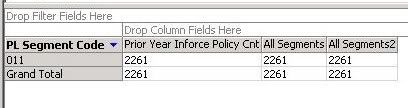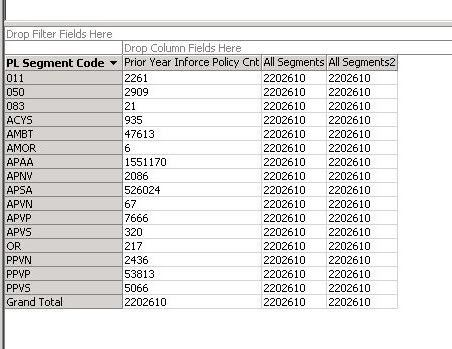#Calculated Measure scenario with a filtered dimensional attribute• ### Question

•I have a cube with a Measure (A) and a dimension (C).  I've created a Calculated Measure (B) whose tuple is Measure A with the all member of Dimension C.  The Calculated Measure looks like this:

([Measures].[A], [C].[ALL])

Scenario 1

When I place the dimension on a pivot table, the Calculated Measure B reflects the correct value, Measure A by all members of dimension C.

Scenario 2

When I place the dimension in the filter area and filter by one or more members, the Calculated Measure B reflects the correct value, Measure A by all members of dimension C.  The same answer as Scenario 1.

Scenario 3

When I place the dimension in the pivot table AND filter by one or more members, I am getting a different answer than I want.  It is filtering first by the members selected and then reflecting the Calculated Measure as the All value of ONLY the selected filter members.  I want the calculated Measure to reflect Measure A by all members of dimension C.  (The same number as in the first two scenarios)

Is there any way to create the Calculated Measure differently so that all three scenarios give me the same answer?

Friday, September 17, 2010 4:09 PM

•You are not using Excel. I can reproduce this issue in OWC11 as well. In fact, different client tools may generate different MDX queries. Excel use subselect as row/column filter, like this:

SELECT NON EMPTY Hierarchize({DrilldownLevel({[Date].[Calendar Year].[All]})}) ON COLUMNS

FROM (SELECT ({[Date].[Calendar Year].&}) ON COLUMNS

FROM [A- Adventure Works DW2008]) WHERE ([Measures].[test])

OWC use a named set with VISUALTOTALS, similar to below one

with

SET [{5366E412-9D07-41F2-9A1A-881DFE55897F}Pivot74Axis1Set0] AS

VISUALTOTALS(

{ [Date].[Calendar Year].[All] ,

[Date].[Calendar Year].&

})

SELECT

NON EMPTY [{5366E412-9D07-41F2-9A1A-881DFE55897F}Pivot74Axis1Set0] ON COLUMNS,

{[Measures].[test]} ON ROWS

The VISUALTOTALS will change the value of [All] member dynamically base on current selection. And the calculated member we used contains the ALL member. So the value is changing in different scenarios. If the calculated member doesn’t contain [All], you will see the same value in above scenarios.

Unfortunately, OWC is being discontinued. It will not be updated in the future. If you want to change this OWC behavior, one workaround could be:

Replace the calculated member:

aggregate(root([PL Segment Dim].[PL Segment Code].[ALL]),[Measures].[Prior Year Inforce Policy Cnt])

with:

aggregate({[PL Segment Dim].[PL Segment Code].[PL Segment Code]},[Measures].[Prior Year Inforce Policy Cnt])

Hope this helps,

Raymond
Raymond Li - MSFT
• Marked as answer by Sunday, September 26, 2010 4:07 AM
Friday, September 24, 2010 5:50 AM

### All replies

•so if I am following you correctly, you did the following...(using Adventure Works 2008R2)

Measures.a = [Measures].[Sales Amount]

Dimension = [Product].[All]

Measures.b = ([Measures].[Sales Amount], [Product].[All])

is this correct? If so, when I follow the same sceanrios as above, I get the same answer always, no matter how I slice it using only the Dimension and Measures.b

so I am not sure how you are getting something different if my logic is correct

FJK
• Proposed as answer by Friday, March 30, 2018 8:39 PM
Friday, September 17, 2010 7:10 PM
•Frank,

Thanks for looking into this.

There is a part I left out.  The calculated measure is actually coded this way.  I am trying to look at the all member of a dimensional attribute within the C dimension called Atta.  Then when I slice the cube on Atta things work fine until I filter on AttA and have it in the pivot table area.  I get a different answer than when Atta is filtered in the Filter Area or when Atta is on the pivot table unfiltered.

([Measures].[A], [C].[AttA].[ALL])

Friday, September 17, 2010 11:51 PM
•Hi Tim,

If you only use [C].[AttA] in your query, you will see the same value.

When you use another dimension, for example: [Product].[Product], the calculated member will return:

([Measures].[A], [C].[AttA].[ALL], [Product].[Product].currentmember)

This is by design, and I think it makes sense.

However, if you just want to return the same value all the time, you can try this:

aggregate(root(),[Measures].[A])

http://technet.microsoft.com/en-us/library/ms146076.aspx

Hope this helps,

Raymond
Raymond Li - MSFT
Monday, September 20, 2010 12:31 PM
•Hi Raymond,

The problem I'm having is that I have the calculated member coded this way:

([Measures].[A], [C].[AttA].[ALL])

but I don't get the same answer.  When I put AttA in the pivot table, and look at each individual member the calculated member shows the ALL level of AttA.  When I put AttA in the filter area and filter by a specific member, the calculated member shows the ALL level of AttA.  But when I put AttA in the pivot table AND filter by a specific member the caclulated member reflects the current member.  I still want it to reflect the ALL level in that last scenario.

Monday, September 20, 2010 5:36 PM
•Hi Raymond,

The problem I'm having is that I have the calculated member coded this way:

But when I put AttA in the pivot table AND filter by a specific member the caclulated member reflects the current member.  I still want it to reflect the ALL level in that last scenario.

This is by design, because SSAS use implicit current context, the expression

([Measures].[A], [C].[AttA].[ALL])

Actually is:

([Measures].[A], [C].[AttA].[ALL], [C].[AttB].[Defaultmember], [D].[**].[Defaultmember]… … )

So if you only use [C].[AttA], you will see the same value, but with other dimensions/attributes, you will see the different values.

If you just want to return the same value all the time, could you can try this:

aggregate(root(),[Measures].[A])

Hope this helps,

Raymond

Raymond Li - MSFT
Tuesday, September 21, 2010 1:50 AM
•Hi Ray,

I'm sorry, I'm not making myself clear.  I would expect for the number to change when I am slicing by other attributes.  But when I put AttA on the pivot table (alone with the Calculated member) and choose a specific member from AttA from the drop down, I am seeing a sliced value rather than the All Value.

Example:

AttA has members X, Y, and Z.  Measure A is 400 for X, 200 for Y and 100 for Z.  When I put Measure B on the pivot table along with AttA,  Initially for Measure B I see 700 for each member, that is what I want and expect.  When I move AttA up to the filter area, I see 700 for Measure B whether I select X, Y, Z or any combination of the three, that is what I want and expect.  When I bring AttA back down to the pivot table and select X from the dropdown, I see X on the pivot table but the value of Measure B is 400.  That is not what I would expect.  If I select X and Y, I see those members on the pivot table and Measure B is 600 for both.  That is not what I would expect.  In either of these scenarios, I would still expect to see 700.

This happens if my browser is Excel 2010 or OWC.  I've also tried it with SSAS 2005 and 2008.

Tuesday, September 21, 2010 3:23 PM
•Hi Tim,

Could you give a try with below expression; can you get the same results in those three scenarios?

aggregate(root([C].[AttA].[ALL]),[Measures].[A])

I tried to reproduce the issue in Excel 2010 64bit/32bit, but it works all the time – I mean the same results in below scenarios:

1)    slicing by [C].[AttA].members

2)    Putting [C].[AttA] in filter pane.

3)    Slicing by [C].[AttA].members but with part of members.

So, could you post two pictures of those two scenarios? I cannot think of a explanation base on your description.

Thanks,

Raymond
Raymond Li - MSFT
• Edited by Wednesday, September 22, 2010 3:20 AM Update Picture
Wednesday, September 22, 2010 3:09 AM
•Hmmm, I am trying the aggregate root syntax and seeing the same exact behavior.  When I filter the member list within the pivot table it is slicing the metric.  Can you post me the MDX for your calculated member?  This is weird.
Wednesday, September 22, 2010 11:51 PM
•Could you post two pictures of scenarios 1 and 2? The calculated member I ‘m using is pretty simply:

CREATE MEMBER CURRENTCUBE.[Measures].[test]

AS ([Measures].[Order Quantity],[Date].[Calendar Year].[All]),

VISIBLE = 1 ,  ASSOCIATED_MEASURE_GROUP = 'Sales' ;

Thanks,

Raymond
Raymond Li - MSFT
Thursday, September 23, 2010 2:06 AM
•Here is the first screen print.  Prior Year Inforce Policy Cnt is the main measure.  The All Segments Calculated Measure is coded this way:

iif

([Measures].[Prior Year Inforce Policy Cnt]=0, NULL, ([Measures].[Prior Year Inforce Policy Cnt], [PL SEGMENT DIM].[PL Segment Code].[All]))

The All Segments2 calculated measure is coded this way:

iif

([Measures].[Prior Year Inforce Policy Cnt]=0, NULL, aggregate(root([PL Segment Dim].[PL Segment Code].[ALL]),[Measures].[Prior Year Inforce Policy Cnt]))

When PL Segment code is placed on the pivot table, the number in All Segments and All Segments2 represents the All Value just as I would expect.The screen shot below occurs when I filter on the PL Segment Code and choose the 011 member.  See how the two calculated members are slicing.  I would expect the number to be the same as in the above screen shot.  It is not displayed here, but if I select a second member from PL Segment Code, the All Segments and All Segments2 calculated measures are reflecting the sum of the two selected members.Thursday, September 23, 2010 4:30 PM
•You are not using Excel. I can reproduce this issue in OWC11 as well. In fact, different client tools may generate different MDX queries. Excel use subselect as row/column filter, like this:

SELECT NON EMPTY Hierarchize({DrilldownLevel({[Date].[Calendar Year].[All]})}) ON COLUMNS

FROM (SELECT ({[Date].[Calendar Year].&}) ON COLUMNS

FROM [A- Adventure Works DW2008]) WHERE ([Measures].[test])

OWC use a named set with VISUALTOTALS, similar to below one

with

SET [{5366E412-9D07-41F2-9A1A-881DFE55897F}Pivot74Axis1Set0] AS

VISUALTOTALS(

{ [Date].[Calendar Year].[All] ,

[Date].[Calendar Year].&

})

SELECT

NON EMPTY [{5366E412-9D07-41F2-9A1A-881DFE55897F}Pivot74Axis1Set0] ON COLUMNS,

{[Measures].[test]} ON ROWS

The VISUALTOTALS will change the value of [All] member dynamically base on current selection. And the calculated member we used contains the ALL member. So the value is changing in different scenarios. If the calculated member doesn’t contain [All], you will see the same value in above scenarios.

Unfortunately, OWC is being discontinued. It will not be updated in the future. If you want to change this OWC behavior, one workaround could be:

Replace the calculated member:

aggregate(root([PL Segment Dim].[PL Segment Code].[ALL]),[Measures].[Prior Year Inforce Policy Cnt])

with:

aggregate({[PL Segment Dim].[PL Segment Code].[PL Segment Code]},[Measures].[Prior Year Inforce Policy Cnt])

Hope this helps,

Raymond
Raymond Li - MSFT
• Marked as answer by Sunday, September 26, 2010 4:07 AM
Friday, September 24, 2010 5:50 AM
•Raymond,

Thank you very much for all of your efforts.  Interestingly, I have some desktops with Excel 2010 where I see the slicing problem and others where I don't.  Not sure what the difference is with the installation but at any rate the workaround you gave me seems to be giving me the correct numbers I'm looking for both in OWC and in Excel.

Thanks again,

Tim

Friday, September 24, 2010 11:38 PM